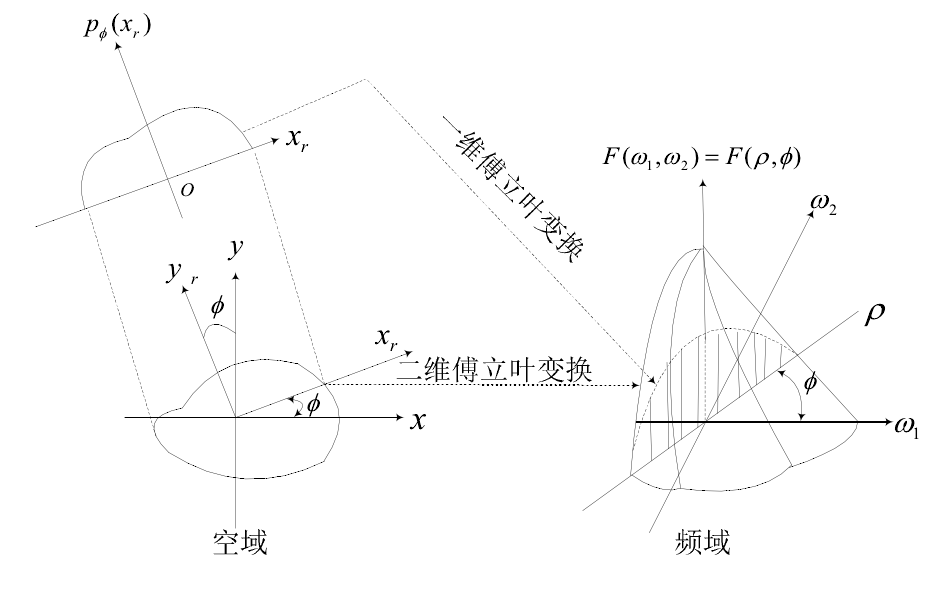# 滤波反投影重建算法实现及应用（matlab）

### 1. 滤波反投影重建算法原理

CT重建算法大致分为解析重建算法和迭代重建算法，随着CT技术的发展，重建算法也变得多种多样，各有各的有特点。本文使用目前应用最广泛的重建算法——滤波反投影算法（FBP）作为模型的基础算法。FBP算法是在傅立叶变换理论基础之上的一种空域处理技术。它的特点是在反投影前将每一个采集投影角度下的投影进行卷积处理，从而改善点扩散函数引起的形状伪影，重建的图像质量较好。### 2. 滤波反投影重建算法过程（以平行束为例）

1. 将原始投影进行一次一维傅立叶变换
2. 设计合适的滤波器，在φ_i的角度下将得到原始投影p(x_r,φ_i)进行卷积滤波，得到滤波后的投影。
3. 将滤波后的投影进行反投影，得到满足x_r=r cos⁡（(θ - φ_i)）方向上的原图像的密度。
4. 将所有反投影进行叠加，得到重建后的投影。

### 3. 滤波器(滤波函数)和内插函数的选取

1. 不准确的数据重建图像就会产生各种伪影。
2. 投影的数据是天然离散的，处理不当的话会产生很大的误差。

### 4. FBP的matlab实现

clc,clear;
%% 各个参数信息
%重建后图片像素个数
M=512;%建议先和接收传感器的个数一样

%旋转的角度 180次旋转
theta=xlsread('angles_180_3.xlsx','Sheet1','a2:a181');

%投影为512行，180列的数据，列的数据对应每一次旋转，512个接收传感器
R=xlsread('Accessory_A.xls','附件3');

%由投影进行反变换
size(R,1);%512

% 设置快速傅里叶变换的宽度
width = 2^nextpow2(size(R,1));

%% 对投影做快速傅里叶变换并滤波
%傅立叶变换
proj_fft = fft(R, width);

% filter 滤波
% R-L是一种基础的滤波算法
filter = 2*[0:(width/2-1), width/2:-1:1]'/width;

% 滤波后结果 proj_filtered
proj_filtered = zeros(width,180);
for i = 1:180
proj_filtered(:,i) = proj_fft(:,i).*filter;
end
figure
subplot(1,2,1),imshow(proj_fft),title('傅立叶变换')
subplot(1,2,2),imshow(proj_filtered),title('傅立叶变换+滤波')

%% 逆快速傅里叶变换并反投影
% 逆快速傅里叶变换 proj_ifft
proj_ifft = real(ifft(proj_filtered));
figure,imshow(proj_ifft),title('逆傅立叶变换')

%反投影到x轴，y轴
fbp = zeros(M); % 假设初值为0
for i = 1:180
rad = theta(i);%弧度， %这个rad 是投影角，不是投影线与x轴夹角，他们之间相差 pi/2
for x = 1:M
for y = 1:M
%{
%最近邻插值法
t = round((x-M/2)*cos(rad)-(y-M/2)*sin(rad));%将每个元素舍X入到最接近的整数。
if t<size(R,1)/2 && t>-size(R,1)/2
fbp(x,y)=fbp(x,y)+proj_ifft(round(t+size(R,1)/2),i);
end
%}
t_temp = (x-M/2) * cos(rad) - (y-M/2) * sin(rad)+M/2  ;
%最近邻插值法
t = round(t_temp) ;
if t>0 && t<=512
fbp(x,y)=fbp(x,y)+proj_ifft(t,i);
end
end
end
end
fbp = (fbp*pi)/180;%512x512 原图像每个像素位置的密度

xlswrite('problem2_origin.xlsx',fbp,'Sheet1');%将得到的重建后的图像数据写入
% 显示结果
figure,imshow(fbp),title('反投影变换后的图像')



fbp算法实现案例

github代码下载 别忘了给star哟~

【1】 范慧赟.CT 图像滤波反投影重建算法的研究[D].硕士学位论文,西北工业大学,2007.
【2】 余晓锷,龚剑,马建华等.CT 原理与技术[M].北京:科学出版社,2013,95-97.
【3】 毛小渊. 二维CT图像重建算法研究[D].南昌航空大学,2016.
【4】 洪虹. CT中金属伪影的校正研究[D].南方医科大学,2013.
【5】 范慧赟. CT图像滤波反投影重建算法的研究[D].西北工业大学,2007.

05-06
08-142万+08-14
04-151万+
05-266417
05-18
11-16
05-302699
08-134万+
07-27
10-31
06-205588
07-031万+
01-044949
©️2020 CSDN 皮肤主题: 成长之路 设计师:Amelia_0503